## Mathematics: Theory

[Note: Page numbers following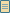indicate pages available in the manuscript viewer]

Throughout the text, Michael uses Hindu-Arabic numerals and the decimal system. Demonstrating a personal mastery of the normal operations of addition, subtraction, multiplication, and division, he provides no discussion of these subjects, perhaps thinking them too simple or obvious to require explanation.

Michael does explain, though, how to multiply, add, divide, and subtract fractions (4b-11b;10a), which he refers to as rutti, or "broken numbers." He always uses fractions to express parts of numbers or remainders. He could not use decimal fractions, because the decimal point had not yet been invented. Where we might write a number as 10.5, Michael would write it as 10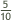.

Later in the text, he discusses radicals (77a-89b) and shows how to operate with square roots and numbers.

Michael also explains the rule of three (10a-11b;10a), perhaps the most basic, most powerful mathematical tool of medieval merchants. According to Michael, this rule could be used not only to solve all problems of commerce, but problems of "all the things in the world." The rule of three states that if four numbers are related to each other such that a is to b as c is to d, then the fourth number can be calculated using the other three. While we understand this as a rule of proportion, Michael did not explain it that way. His concern centered on the ordering of the numbers so that the proper calculations could take place. In the text, he almost always states his problems so that d is the unknown, and therefore the solution will be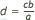. (Learn more about Michael's use of the rule of three.)

Surprisingly, Michael gives no explanation of the rule of double false position, which was one of the most ingenious techniques of the Middle Ages and one of Michael's personal favorites. The method involves asking the user to come up with two arbitrary, incorrect solutions about the value of an unknown, then manipulating the result to get the right answer. The technique allowed Michael to solve problems that we would now call linear equations, at a time when the absence of modern notation made them difficult. (Learn more about Michael's use of the rule of double false position.)

Algebra gets extensive treatment from Michael (12a-18a, 16b-17b;12a). He begins by giving credit for its invention to "Alzibran, a Saracen with same name, that is alzebra." He was right about algebra's Arabic origins, but wrong about the name. The first known treatise on algebra was written by a ninth-century Arabic scholar named Mohammed ibn al-Khwarizmi. The words al-jabr, which were part of the title of Khwarizmi's work, were translated as "algebra" in medieval Latin and became a standard word for the subject. In Michael's time, however, algebra was most often referred to as the regola della cosa. The word cosa generally means "thing," but in this context, it means "the thing to be found" or "the unknown." Algebra was therefore usually called the "rule of the unknown."

Michael starts his discussion of algebra with a list of six first-order and second-order equations, ranging (in modern notation) from ax = n to ax + n = b. He proceeds to explain them, then solve problems illustrating their use. Later he gives a list of 18 more complicated higher-order equations, ranging from c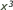= n to d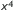+ b= n.

Michael wrote all these equations out in words and sentences because modern notation had not yet been invented. Even such basic signs as = (equals), + (plus), x (times), and - (minus) did not yet exist. One symbol Michael did use was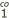, which he used where we would use the variable x. But an unknown likehad to be written in words like zenso di zenso, while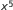would be written chubo di zensi (zenso means "square"). (Learn more about Michael's use of algebra.)